SNAP Library 6.0, Developer Reference  2020-12-09 16:24:20 SNAP, a general purpose, high performance system for analysis and manipulation of large networks
TBPGraph::TEdgeI Class Reference

Edge iterator. Only forward iteration (operator++) is supported. More...

`#include <graph.h>`

Collaboration diagram for TBPGraph::TEdgeI: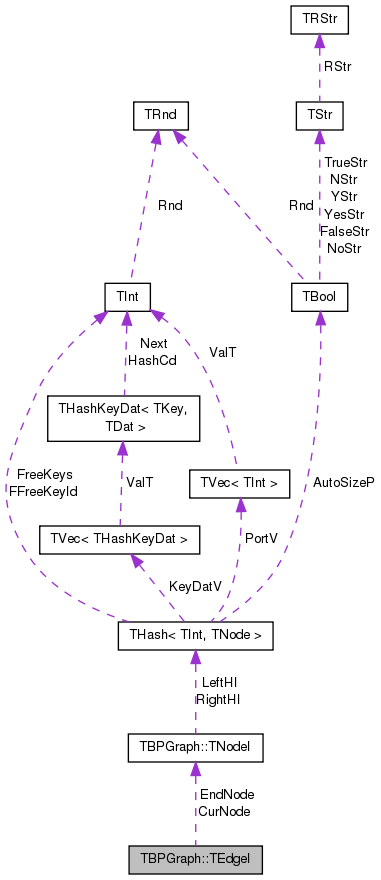[legend]

## Public Member Functions

TEdgeI ()

TEdgeI (const TNodeI &NodeI, const TNodeI &EndNodeI, const int &EdgeN=0)

TEdgeI (const TEdgeI &EdgeI)

TEdgeIoperator= (const TEdgeI &EdgeI)

TEdgeIoperator++ (int)
Increment iterator. More...

bool operator< (const TEdgeI &EdgeI) const

bool operator== (const TEdgeI &EdgeI) const

int GetId () const
Gets edge ID. Always returns -1 since only edges in multigraphs have explicit IDs. More...

int GetSrcNId () const
Gets the source ('left' side) of an edge. Since the graph is undirected this is the node with smaller ID of the edge endpoints. More...

int GetDstNId () const
Gets destination ('right' side) of an edge. Since the graph is undirected this is the node with greater ID of the edge endpoints. More...

int GetLNId () const
Gets the ID of the node on the 'left' side of the edge. More...

int GetRNId () const
Gets the ID of the node on the 'right' side of the edge. More...

TNodeI CurNode

TNodeI EndNode

int CurEdge

class TBPGraph

## Detailed Description

Edge iterator. Only forward iteration (operator++) is supported.

Definition at line 1018 of file graph.h.

## Constructor & Destructor Documentation

 TBPGraph::TEdgeI::TEdgeI ( )
inline

Definition at line 1023 of file graph.h.

1023 : CurNode(), EndNode(), CurEdge(0) { }
TNodeI CurNode
Definition: graph.h:1020
TNodeI EndNode
Definition: graph.h:1020
 TBPGraph::TEdgeI::TEdgeI ( const TNodeI & NodeI, const TNodeI & EndNodeI, const int & EdgeN = `0` )
inline

Definition at line 1024 of file graph.h.

1024 : CurNode(NodeI), EndNode(EndNodeI), CurEdge(EdgeN) { }
TNodeI CurNode
Definition: graph.h:1020
TNodeI EndNode
Definition: graph.h:1020
 TBPGraph::TEdgeI::TEdgeI ( const TEdgeI & EdgeI )
inline

Definition at line 1025 of file graph.h.

1025 : CurNode(EdgeI.CurNode), EndNode(EdgeI.EndNode), CurEdge(EdgeI.CurEdge) { }
TNodeI CurNode
Definition: graph.h:1020
TNodeI EndNode
Definition: graph.h:1020

## Member Function Documentation

 int TBPGraph::TEdgeI::GetDstNId ( ) const
inline

Gets destination ('right' side) of an edge. Since the graph is undirected this is the node with greater ID of the edge endpoints.

Definition at line 1037 of file graph.h.

References TBPGraph::TNodeI::GetOutNId().

1037 { return CurNode.GetOutNId(CurEdge); }
int GetOutNId(const int &NodeN) const
Returns ID of NodeN-th out-node (the node the current node points to).
Definition: graph.h:1005
TNodeI CurNode
Definition: graph.h:1020

Here is the call graph for this function: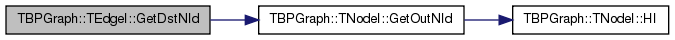Here is the caller graph for this function: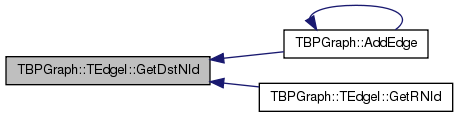int TBPGraph::TEdgeI::GetId ( ) const
inline

Gets edge ID. Always returns -1 since only edges in multigraphs have explicit IDs.

Definition at line 1033 of file graph.h.

1033 { return -1; }
 int TBPGraph::TEdgeI::GetLNId ( ) const
inline

Gets the ID of the node on the 'left' side of the edge.

Definition at line 1039 of file graph.h.

References GetSrcNId().

1039 { return GetSrcNId(); }
int GetSrcNId() const
Gets the source ('left' side) of an edge. Since the graph is undirected this is the node with smaller...
Definition: graph.h:1035

Here is the call graph for this function: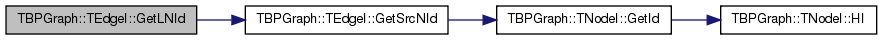int TBPGraph::TEdgeI::GetRNId ( ) const
inline

Gets the ID of the node on the 'right' side of the edge.

Definition at line 1041 of file graph.h.

References GetDstNId().

1041 { return GetDstNId(); }
int GetDstNId() const
Gets destination ('right' side) of an edge. Since the graph is undirected this is the node with great...
Definition: graph.h:1037

Here is the call graph for this function: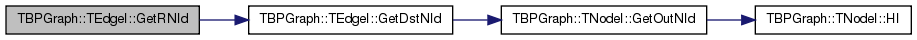int TBPGraph::TEdgeI::GetSrcNId ( ) const
inline

Gets the source ('left' side) of an edge. Since the graph is undirected this is the node with smaller ID of the edge endpoints.

Definition at line 1035 of file graph.h.

References TBPGraph::TNodeI::GetId().

1035 { return CurNode.GetId(); }
int GetId() const
Returns ID of the current node.
Definition: graph.h:989
TNodeI CurNode
Definition: graph.h:1020

Here is the call graph for this function: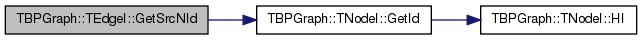Here is the caller graph for this function: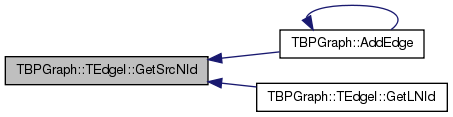TEdgeI& TBPGraph::TEdgeI::operator++ ( int )
inline

Increment iterator.

Definition at line 1028 of file graph.h.

References TBPGraph::TNodeI::GetOutDeg().

1028  { CurEdge++; if (CurEdge >= CurNode.GetOutDeg()) { CurEdge=0; CurNode++;
1029  while (CurNode < EndNode && CurNode.GetOutDeg()==0) { CurNode++; } } return *this; }
int GetOutDeg() const
Returns out-degree of the current node (returns same as value GetDeg() since the graph is undirected)...
Definition: graph.h:999
TNodeI CurNode
Definition: graph.h:1020
TNodeI EndNode
Definition: graph.h:1020

Here is the call graph for this function: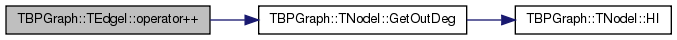bool TBPGraph::TEdgeI::operator< ( const TEdgeI & EdgeI ) const
inline

Definition at line 1030 of file graph.h.

References CurEdge, and CurNode.

1030 { return CurNode<EdgeI.CurNode || (CurNode==EdgeI.CurNode && CurEdge<EdgeI.CurEdge); }
TNodeI CurNode
Definition: graph.h:1020
 TEdgeI& TBPGraph::TEdgeI::operator= ( const TEdgeI & EdgeI )
inline

Definition at line 1026 of file graph.h.

References CurEdge, CurNode, and EndNode.

1026 { if (this!=&EdgeI) { CurNode=EdgeI.CurNode; EndNode=EdgeI.EndNode; CurEdge=EdgeI.CurEdge; } return *this; }
TNodeI CurNode
Definition: graph.h:1020
TNodeI EndNode
Definition: graph.h:1020
 bool TBPGraph::TEdgeI::operator== ( const TEdgeI & EdgeI ) const
inline

Definition at line 1031 of file graph.h.

References CurEdge, and CurNode.

1031 { return CurNode == EdgeI.CurNode && CurEdge == EdgeI.CurEdge; }
TNodeI CurNode
Definition: graph.h:1020

## Friends And Related Function Documentation

 friend class TBPGraph
friend

Definition at line 1042 of file graph.h.

## Member Data Documentation

 int TBPGraph::TEdgeI::CurEdge
private

Definition at line 1021 of file graph.h.

Referenced by operator<(), operator=(), and operator==().

 TNodeI TBPGraph::TEdgeI::CurNode
private

Definition at line 1020 of file graph.h.

Referenced by operator<(), operator=(), and operator==().

 TNodeI TBPGraph::TEdgeI::EndNode
private

Definition at line 1020 of file graph.h.

Referenced by operator=().

The documentation for this class was generated from the following file: